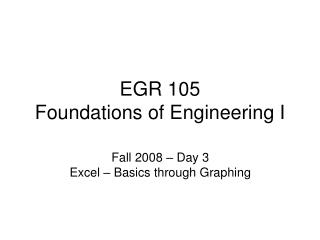# EGR 105 Foundations of Engineering I - PowerPoint PPT PresentationDownload PresentationEGR 105 Foundations of Engineering I

Presentation Description
Download Presentation## EGR 105 Foundations of Engineering I

- - - - - - - - - - - - - - - - - - - - - - - - - - - E N D - - - - - - - - - - - - - - - - - - - - - - - - - - -
##### Presentation Transcript

1. EGR 105 Foundations of Engineering I Fall 2008 – Day 3 Excel – Basics through Graphing

2. Time Management • Timesheet #2 due today (#1???) • Civil Engineering reports due next Wednesday, Oct 1 • Today’s assignment due Oct 7 • Can also work on it next week, Oct 1 • Work on your presentations……

3. EGR105 – Day 3 Topics • Excel basics • Cell referencing • Math functions • Graphing • Homework Assignment

4. Excel Basics • Workbook organization • Cells (row/col) • Worksheets (names) • Entering and formatting data • Copy & paste • Fill • Simple math • +, –, *, /, ^ • Entry starts with = • “autosum” • Relative copy for math operations • Data sort • Split window

5. Referring to Cells • Standard way: row column notation • Actually, letter number • For fixed, add \$s (“absolute cell reference”) • Cell names – can “rename” cells by changing content of box on upper left • Useful for formulas

6. More Complicated Math • What if you had to compute or n = number of points

7. Built-in Functions in Excel • Syntax: • Entry always starts with an equal sign (=) • Next is the name of the function followed by parentheses containing parameters or values to be operated on (arguments) • Examples: = SUM ( A10:C20 ) = SQRT ( B17 ) • Many types available

8. Some work on single arguments, some on groups of arguments, some on none • Composition of functions works: = SQRT ( SUM ( A10:B17 ) ) • How to invoke: • Know the name of the function • Function wizard button: f x

9. Elementary Math = SQRT (x) = ABS (x) = FACT (x) = SUM (x1,x2,…) = GCD (x1,x2,…) = LCM (x1,x2,…) = CEILING (x1,x2,…) = ROUND (x) = SUM (x1,x2,…) = COUNT (x1,x2,…) = EXP (x) = LOG (x) = LOG10 (x) = POWER (x,y) = RAND ( ) …

10. Trigonometry = PI ( ) = DEGREES (x) = RADIANS (x) = SIN (x) = COS (x) = TAN (x) = ACOS (x) = SINH (x) = ATAN2 (x,y) …

11. Logical Functions • If test: = IF ( logical test, value if true, value if false ) • Example: = IF (A10 >= B20,A10,0) • Others: AND, NOT, OR

12. Statistics = min (c1:d10) = max (c1:d10) = average (c1:d10) = median (c1:d10) = stdev (c1:d10) = var (c1:d10)

13. Interfacing to the World • Importing/Exporting data • “open” • “save as”

14. Graphics • Excel’s terminology: • “Chart” • Engineers commonly use XY scatter, not line!! • “Category” is the independent variable, horizontal axis, abscissa • “Value” is the dependent variable, vertical axis, ordinate • Excel’s plot wizard

15. Graphing Standards • Symbolism: • Experimental data • Theoretical curves • Labeling: • Axes names • Units • Types of scales: • Linear • Range to show • Semilog & log • Multiple curves on one set of axes • Legends

16. Homework Assignment See passed out sheet or course web site